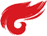简单售货机代码

#define _CRT_SCUER_WARINGS
#include<iostream>
using namespace std;
class Goods {

public:
Goods()
{
weight = 0;
next = NULL;
cout << "创建了一个重量为" << weight << "货物" << endl;

}
Goods(int w) {
weight = w;
next = NULL;
total_weight += weight;
cout << "创建了一个重量为" << weight <<"货物" <<endl;
cout << "当前厂库量为:" << GetTotal_Weight() << endl;
}
~Goods()
{
cout << "卖出了一箱重量是" << weight << "货物" << endl;
total_weight -= weight;
cout << "当前厂库量为:" << GetTotal_Weight() << endl;
}
static int GetTotal_Weight() {

}
{
{
cout << "库中已没有货物" << endl;
}
else
{
delete temp;
}
}
Goods *new_goods = new Goods(w);
{
}
else
{
}

}

Goods * next;
private:

int weight;

static int total_weight;

};
int Goods::total_weight = 0;

int main()
{
int flag = 0;
do
{
cout << "1 进货" << endl;
cout << "2 出货" << endl;
cout << "3 退出" << endl;
cin >> flag;
switch (flag)
{
case 1:cout << "请输入要创建货物的重量" << endl;
cin >> flag;
break;
break;
case 3:
return 0;

default:
break;
}

} while (true);

//system("pause");
return 0;
}

posted on 2018-08-04 16:02  幽love梦费重寻  阅读(288)  评论(0编辑  收藏  举报